# 1、柱状图中最大的矩形

输入: [2,1,5,6,2,3]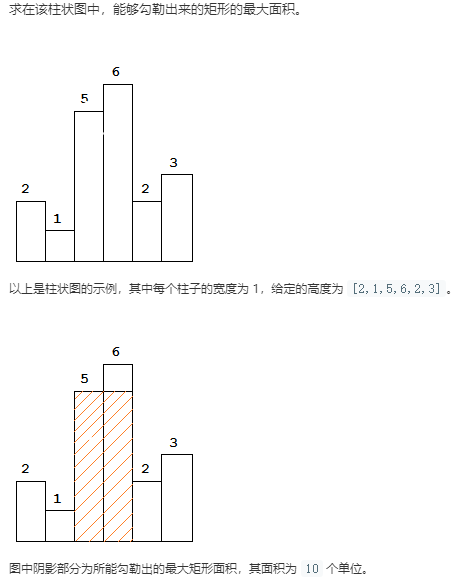## 1.1、解题思路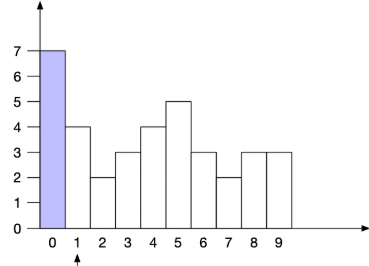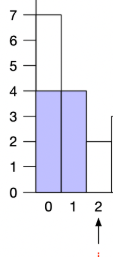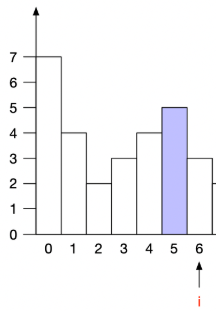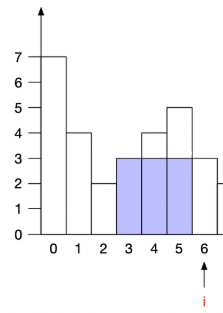## 1.2、算法

public int largestRectangleArea(int[] heights) {
Stack<Integer> stack = new Stack<>();
stack.push(-1);
int maxArea = 0;
for (int i = 0; i < heights.length; i++) {
// （-1肯定不会考虑了，因为是负数，-1只是起一个占位的作用），这样就保证了栈中最少有2个元素。
//获取栈顶元素，当栈顶元素不是第一个元素 -1 且数组准备呈下降关系时，我们开始从栈中取出元素同时计算最大面积
while (stack.peek() != -1 && heights[i] < heights[stack.peek()]) {
// 将栈中的序号弹出，作为高度。而宽度 =  当前位置 - 刚刚出栈后栈现在的元素 -1
maxArea = Math.max(heights[stack.pop()] * (i - stack.peek() - 1), maxArea);
}
stack.push(i);
}
//当上面的遍历完成，最后一个元素无论如何也不会加入到最大面积的计算中，这个时候的宽度我们要向后取一位。再进行计算，知道栈中只剩下-1
while (stack.peek() != -1) {
maxArea = Math.max(heights[stack.pop()] * (heights.length - stack.peek() - 1), maxArea);
}
return maxArea;
}


## 1.3、测试

    @Test
public void test(){
int[] matrix = new int[]{7,4,2,3,4,5,3,2,3,3};
// int[] matrix = new int[]{1,2,3,4,5};

System.out.println(largestRectangleArea(matrix));
}